# Percentages + multiplication principle - math problems

#### Number of problems found: 12

• An aircraft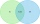An aircraft manufacturing company has submitted bids on two separate airline contracts, A and B. The company feels that it has a 70% chance of winning contract A and a 25% chance of winning contract B. Furthermore, it believes that winning contract A is i
• Created trioWhat is the probability that in the created trio, which consists of 19 boys and 12 girls, they will be: a) the boys themselves b) the girls themselves c) 2 boys and 1 girl?
• Three colors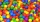Find the probability that 3 balls of the same color will be drawn from fate with 10 white, 10 red, and 10 blue balls.
• Hazard game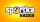In the Sportka hazard game, 6 numbers out of 49 are drawn. What is the probability that we will win: a) second prize (we guess 5 numbers correctly) b) the third prize (we guess 4 numbers correctly)?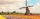How large must the group of people be so that the probability that two people have a birthday on the same day of the year is greater than 90%?
• The testThe test contains four questions, and there are five different answers to each of them, of which only one is correct, the others are incorrect. What is the probability that a student who does not know the answer to any question will guess the right answer
• The universityAt a certain university, 25% of students are in the business faculty. Of the students in the business faculty, 66% are males. However, only 52% of all students at the university are male. a. What is the probability that a student selected at random in the
• Two acesFrom a 32 card box we randomly pick 1 card and then 2 more cards. What is the probability that last two drawn cards are aces?
• ThrowWe throw 2 times with 2 dices. What is the probability that the first roll will fall more than sum of 9 and the second throw have sum 3 or does not have the sum 4?
• travel agency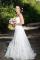Small travel agency offers 5 different tours at honeymoon. What is the probability that the bride and groom choose the same tour (they choose independently)?
• Green - Red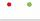We have 5 bags. Each consist one green and 2 red balls. From each we pull just one ball. What is the probability that we doesn't pull any green ball?
• Events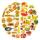Event P has probability of 0.84. What is the probability that the event P occurs in 3, 5, 7 try.

We apologize, but in this category are not a lot of examples.
Do you have an exciting math question or word problem that you can't solve? Ask a question or post a math problem, and we can try to solve it.

We will send a solution to your e-mail address. Solved examples are also published here. Please enter the e-mail correctly and check whether you don't have a full mailbox.

Our percentage calculator will help you quickly calculate various typical tasks with percentages. Percentages Problems. Multiplication principle - math problems.